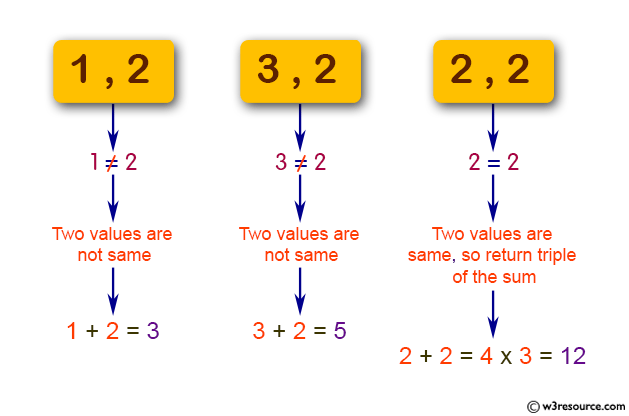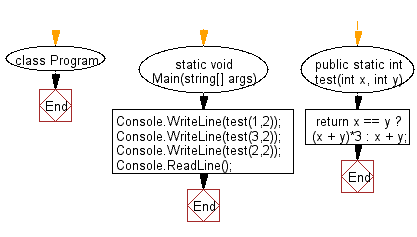﻿ C# - Compute the sum of the two given integer values# C# Sharp Basic Algorithm Exercises: Compute the sum of the two given integer values

## C# Sharp Basic Algorithm: Exercise-1 with Solution

Write a C# Sharp program to compute the sum of the two given integer values. If the two values are the same, then return triple their sum.

Pictorial Presentation:Sample Solution:

C# Sharp Code:

``````using System;
namespace exercises
{
class Program
{
static void Main(string[] args)
{
Console.WriteLine(test(1,2));
Console.WriteLine(test(3,2));
Console.WriteLine(test(2,2));
}

public static int test(int x, int y)
{
return x == y ? (x + y)*3 : x + y;
}
}
}
```
```

Sample Output:

```3
5
12```

Flowchart:C# Sharp Code Editor:

Improve this sample solution and post your code through Disqus

What is the difficulty level of this exercise?

Test your Programming skills with w3resource's quiz.

﻿